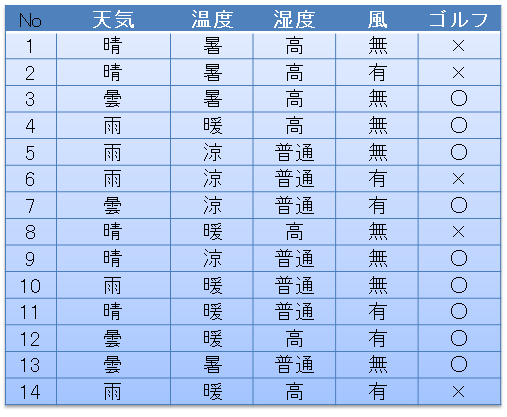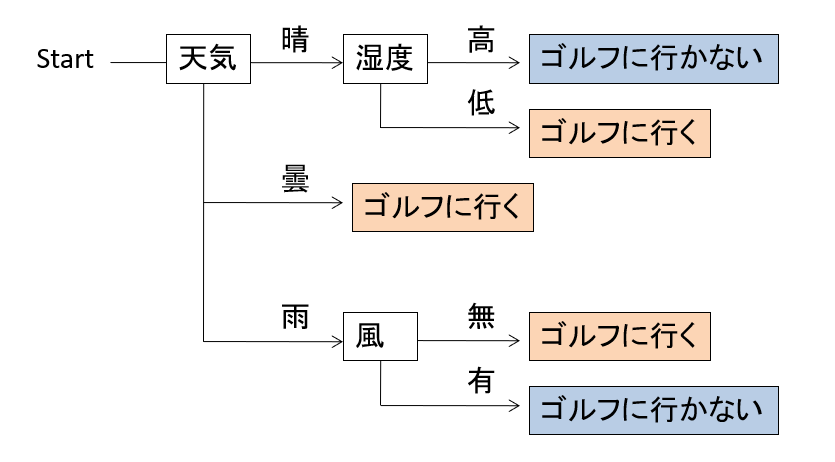# What is a decision tree?

### Example of decision tree

For example, if you have the following data: When the weather, temperature, humidity, and wind are as follows, the day you went to golf is marked with 〇, and the day you did not go is marked with x. Suppose you have 14 such data.From this data, when do you go golf? The one described as a tree structure rule as shown below is called a decision tree or decision tree.For example, if you look at this decision tree from the start, first check the weather, then check the humidity in it if it is fine, go to golf if it is high, and not if it is low. Also, when you go back to the beginning and the weather is cloudy, you can think of going golf, regardless of other conditions.

An algorithm that automatically creates such a decision tree from data is known. In this article, we will implement an algorithm called ID3 from among them using python.

### Academic position of decision tree

Decision trees belong to supervised learning and classification learning, mainly in machine learning, which is part of AI. This classification learning is a general term for a method in which learning data with correct answer data is given and a model that derives the correct answer is automatically created from it. Deep learning [^ 1], which has achieved good results in image recognition, which has become popular in recent years, is also a type of classification learning. The difference between deep learning and decision trees is whether or not the generated rules are human-understandable. Unlike deep learning, which is said to give an answer but the reason is unknown to humans, the rules are easy to understand as in the example of the decision tree above, so create a program that automatically finds the answer simply as classification learning. Not only that, decision tree generation algorithms are also used from the perspective of data mining, such as creating decision trees to help people understand data.

# About algorithm ID3 to be created

ID3 [^ 2] is a decision tree generation algorithm invented by Ross Quinlan in 1986. It has the following features.

1. We only deal with labeled data such as going / not going golf, which is called categorical data (nominal scale). Numerical data cannot be handled.
2. Using an index called information entropy, search for the attribute (the column of data) that has the smallest variation in the class attribute value (the column you want to classify).

### Handling of numerical data

In the case of the algorithm called C4.5 [^ 3], which is an extension of ID3, it is possible to classify numerical data, but since the basic idea is the same as ID3, ID3 will be taken up first in this article. ..

# Development environment

The program shown below has been confirmed to work in the following environment.

--jupyter notebooks (I used azure notebooks)

• Python 3.6 --import library: math, pandas, functools (do not use scikit-learn, tensorflow, etc.)

# Full program

For the time being, copy the program to Jupyter Notebook and it will work.

#### `id3.py`

``````
import math
import pandas as pd
from functools import reduce

#data set
d = {
"weather":["Fine","Fine","Cloudy","rain","rain","rain","Cloudy","Fine","Fine","rain","Fine","Cloudy","Cloudy","rain"],
"temperature":["Hot","Hot","Hot","Warm","Ryo","Ryo","Ryo","Warm","Ryo","Warm","Warm","Warm","Hot","Warm"],
"Humidity":["High","High","High","High","usually","usually","usually","High","usually","usually","usually","High","usually","High"],
"Wind":["Nothing","Yes","Nothing","Nothing","Nothing","Yes","Yes","Nothing","Nothing","Nothing","Yes","Yes","Nothing","Yes"],
#The last column is the data that you want to derive from other columns, also called the objective variable and correct answer data.
"golf":["×","×","○","○","○","×","○","×","○","○","○","○","○","×"],
}
df0 = pd.DataFrame(d)

#Lambda expression for value distribution, argument is pandas.Series, return value is an array containing the number of each value
cstr = lambda s:[k+":"+str(v) for k,v in s.value_counts().items()]

#Decision tree data structure, name:The name of this node (trunk), df:Data associated with this node,
# edges:In the case of a leaf node with no edges below the list of edges (branches) coming out of this node, edges are an empty array.
tree = {"name":"decision tree "+df0.columns[-1]+" "+str(cstr(df0.iloc[:,-1])),"edges":[],"df":df0}

#Tree generation saves the trunks that may be able to generate branches in this opn and examines them in order.
#When adding a trunk to opn, it is added at the end of the array, and the node to be investigated is extracted from the beginning of opn, so it is breadth-first search.
opn = [tree]

#Lambda expression to calculate entropy, argument is pandas.Series, return value is entropy value
entropy = lambda s:-reduce(lambda x,y:x+y,map(lambda x:(x/len(s))*math.log2(x/len(s)),s.value_counts()))

#Repeat until opn is empty.
while(len(opn)!=0):
#Extract the beginning of opn and extract the data held by that node.
node1 = opn.pop(0)
df1 = node1["df"]
#If the entropy of this node is 0, the edge cannot be expanded any more, so the search for this node ends.
if 0==entropy(df1.iloc[:,-1]):
continue
#Create a variable to save the list of branchability attribute values.
attrs = {}
#Examine all attributes except the last column of class attributes.
for attr in list(df1)[:-1]:
#Create a variable to save the entropy when branching with this attribute, the data after branching, and the attribute value to branch.
attrs[attr] = {"entropy":0,"dfs":[],"values":[]}
#Investigate all possible values for this attribute.
for value in set(df1[attr]):
#Filter the data by attribute value.
df2 = df1[df1[attr]==value]
#Calculate the entropy and save the related data and values respectively.
attrs[attr]["entropy"] += entropy(df2.iloc[:,-1])*len(df2)/len(df1)
attrs[attr]["dfs"] += [df2]
attrs[attr]["values"] += [value]
pass
pass
#If there is no attribute that can separate the class value, the investigation of this node is terminated.
if len(attrs)==0:
continue
#Get the attribute that minimizes entropy.
attr = min(attrs,key=lambda x:attrs[x]["entropy"])
#Add the value of each branching attribute and the data after branching to the tree and opn, respectively.
for d,v in zip(attrs[attr]["dfs"],attrs[attr]["values"]):
tree2 = {"name":attr+"="+v,"edges":[],"df":d.drop(columns=attr)}
node1["edges"].append(tree2)
opn.append(tree2)
pass

#Output the dataset.
print(df0,"\n-------------")
#Method to convert tree to characters, argument is tree:Tree data structure, indent:Indentation when expressing characters,
#The return value is a character representation of tree. This method is called recursively to convert everything in the tree to characters.
def tstr(tree,indent=""):
#Create a character representation for this node.
s = indent+tree["name"]+str(cstr(tree["df"].iloc[:,-1]) if len(tree["edges"])==0 else "")+"\n"
#Loop on all edges from this node.
for e in tree["edges"]:
#Add the character representation of the child node to the character representation of this node.
s += tstr(e,indent+"  ")
pass
return s
#Characterize the tree and output it.
print(tstr(tree))
``````

### Execution result

When executed, the decision tree is output as a character notation.

``````decision tree golf
weather=Fine
Humidity=High['×:3']
Humidity=usually['○:2']
weather=rain
Wind=Yes['×:2']
Wind=Nothing['○:3']
weather=Cloudy['○:4']
``````

### Change the attribute (data column) you want to learn

The last column of data d is the class attribute (the data column you want to classify).

#### `data.py`

``````
d = {
"weather":["Fine","Fine","Cloudy","rain","rain","rain","Cloudy","Fine","Fine","rain","Fine","Cloudy","Cloudy","rain"],
"temperature":["Hot","Hot","Hot","Warm","Ryo","Ryo","Ryo","Warm","Ryo","Warm","Warm","Warm","Hot","Warm"],
"Humidity":["High","High","High","High","usually","usually","usually","High","usually","usually","usually","High","usually","High"],
"golf":["×","×","○","○","○","×","○","×","○","○","○","○","○","×"],
#The last attribute is the class attribute (the attribute that is the object of classification)
"Wind":["Nothing","Yes","Nothing","Nothing","Nothing","Yes","Yes","Nothing","Nothing","Nothing","Yes","Yes","Nothing","Yes"],
}
``````

For example, if you run the wind last as above, you will get the following result:

``````decision tree wind['Nothing:8', 'Yes:6']
golf=×
weather=rain['Yes:2']
weather=Fine
temperature=Hot
Humidity=High['Nothing:1', 'Yes:1']
temperature=Warm['Nothing:1']
golf=○
weather=Cloudy
temperature=Hot['Nothing:2']
temperature=Ryo['Yes:1']
temperature=Warm['Yes:1']
weather=Fine
temperature=Ryo['Nothing:1']
temperature=Warm['Yes:1']
weather=rain['Nothing:3']
``````

When there is wind / no wind, rules are created such as not going to golf, branching first when going.